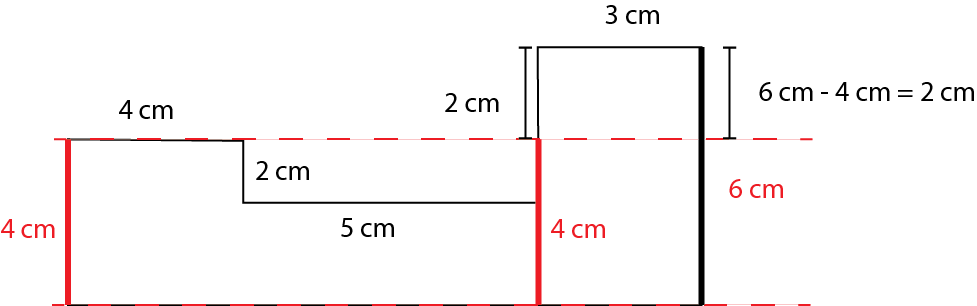# Perimeter of Shapes

The perimeter of a shape means how much distance you cover when you walk around it.

We highly recommend that you take a look at the link we provided. After reading it, understanding this topic will become much simpler.

What is to be measured is a length, so we need to measure it in units of length. If you're using the metric system, it would be in meters (m, dm, cm, etc.) if you're using the imperial system it would be in miles, feet, inches etc.

To calculate the perimeter of a shape, you need to add up all the side lengths. This is because you need to find out how much you've walked in total when you move along the edges from the point you've determined and come back to where you started.

Hope you get the basic idea.

To calculate the perimeter length of a shape, all edge lengths either need to be known or must be determinable from the given information.

You can find their lengths by transfering the sides.

# Example

Work out the perimeter of the shape.

We will calculate the perimeter of the shape, but we need to find the sides that are not given.

12 cm = 4 cm + 3 cm + missing length

Therefore, if we subtract the total of the given parts, which is 7 cm, from 12 cm, we find that the length of the missing part is 5 cm.

Let's also find the other side that hasn't been given.# Representing the Perimeter Mathematically

Perimeter = P
Side = s , a, b, c , x , y, z , l , w exct..

When expressing the perimeter, we don't always use the word 'perimeter'; we often simply write 'P', such as P = 40 cm. This means the perimeter of the shape is 40 cm. The edges of the shape are line segments, and we represent these line segments with lowercase letters (in mathematics, uppercase letters represent points). While we often use expressions like x, y, z, we also use other letters like a, b, c, or even d. For rectangles, we might use 'l' for the longer side ( length ) and 'w' for the shorter side ( width ) .

# Perimeter of Regular Polygons

Finding the perimeter of regular polygons is quite easy because all side lengths are equal.

For example, if the side length of a hexagon is 10 cm, the total perimeter = 10 cm + 10 cm + 10 cm + 10 cm + 10 cm + 10 cm = 60 cm. Alternatively, we can calculate it as 10 cm x 6 = 60 cm.